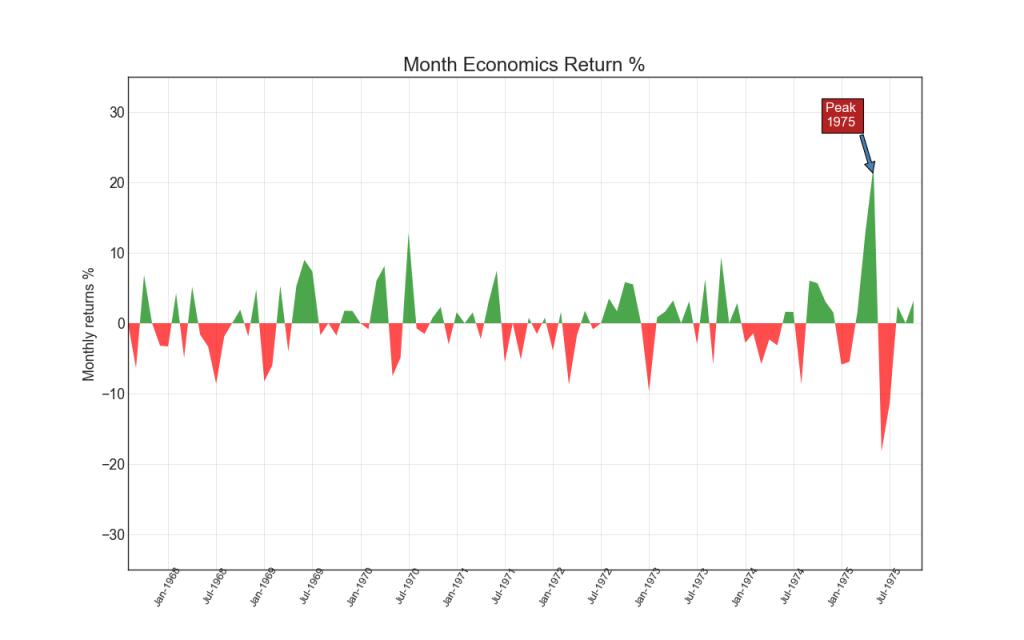# Python Data Visualisation for Business Analyst – How to plot Area Chart in Python

In this data visualisation tutorial, you will learn How to plot Area Chart in Python.

By coloring the area between the axis and the lines, the area chart throws more emphasis not just on the peaks and troughs but also the duration of the highs and lows. The longer the duration of the highs, the larger is the area under the line.

## Setup

Run this once before the plot’s code. The individual charts, however, may redefine its own aesthetics.

``````/* !pip install brewer2mpl */
import numpy as np
import pandas as pd
import matplotlib as mpl
import matplotlib.pyplot as plt
import seaborn as sns
import warnings; warnings.filterwarnings(action='once')

large = 22; med = 16; small = 12
params = {'axes.titlesize': large,
'legend.fontsize': med,
'figure.figsize': (16, 10),
'axes.labelsize': med,
'axes.titlesize': med,
'xtick.labelsize': med,
'ytick.labelsize': med,
'figure.titlesize': large}
plt.rcParams.update(params)
plt.style.use('seaborn-whitegrid')
sns.set_style("white")
%matplotlib inline

/* Version */
print(mpl.__version__)
print(sns.__version__)``````
Data: economics

## How to plot Area Chart in Python

``````import numpy as np
import pandas as pd

/* Prepare Data */
x = np.arange(df.shape)
y_returns = (df.psavert.diff().fillna(0)/df.psavert.shift(1)).fillna(0) * 100

/* Plot */
plt.figure(figsize=(16,10), dpi= 80)
plt.fill_between(x[1:], y_returns[1:], 0, where=y_returns[1:] >= 0, facecolor='green', interpolate=True, alpha=0.7)
plt.fill_between(x[1:], y_returns[1:], 0, where=y_returns[1:] <= 0, facecolor='red', interpolate=True, alpha=0.7)

/* Annotate */
plt.annotate('Peak \n1975', xy=(94.0, 21.0), xytext=(88.0, 28),
bbox=dict(boxstyle='square', fc='firebrick'),
arrowprops=dict(facecolor='steelblue', shrink=0.05), fontsize=15, color='white')

/* Decorations */
xtickvals = [str(m)[:3].upper()+"-"+str(y) for y,m in zip(df.date.dt.year, df.date.dt.month_name())]
plt.gca().set_xticks(x[::6])
plt.gca().set_xticklabels(xtickvals[::6], rotation=90, fontdict={'horizontalalignment': 'center', 'verticalalignment': 'center_baseline'})
plt.ylim(-35,35)
plt.xlim(1,100)
plt.title("Month Economics Return %", fontsize=22)
plt.ylabel('Monthly returns %')
plt.grid(alpha=0.5)
plt.show()``````# Personal Career & Learning Guide for Data Analyst, Data Engineer and Data Scientist

## Applied Machine Learning & Data Science Projects and Coding Recipes for Beginners

A list of FREE programming examples together with eTutorials & eBooks @ SETScholars

# Projects and Coding Recipes, eTutorials and eBooks: The best All-in-One resources for Data Analyst, Data Scientist, Machine Learning Engineer and Software Developer

Topics included: Classification, Clustering, Regression, Forecasting, Algorithms, Data Structures, Data Analytics & Data Science, Deep Learning, Machine Learning, Programming Languages and Software Tools & Packages.
(Discount is valid for limited time only)`Disclaimer: The information and code presented within this recipe/tutorial is only for educational and coaching purposes for beginners and developers. Anyone can practice and apply the recipe/tutorial presented here, but the reader is taking full responsibility for his/her actions. The author (content curator) of this recipe (code / program) has made every effort to ensure the accuracy of the information was correct at time of publication. The author (content curator) does not assume and hereby disclaims any liability to any party for any loss, damage, or disruption caused by errors or omissions, whether such errors or omissions result from accident, negligence, or any other cause. The information presented here could also be found in public knowledge domains.`

# Learn by Coding: v-Tutorials on Applied Machine Learning and Data Science for Beginners

Please do not waste your valuable time by watching videos, rather use end-to-end (Python and R) recipes from Professional Data Scientists to practice coding, and land the most demandable jobs in the fields of Predictive analytics & AI (Machine Learning and Data Science).

The objective is to guide the developers & analysts to “Learn how to Code” for Applied AI using end-to-end coding solutions, and unlock the world of opportunities!

Python Examples for Beginners: Python Code to Calculate the Area of a Triangle

How to create bar chart in R

Statistics for Beginners – Introduction to Excel Charts